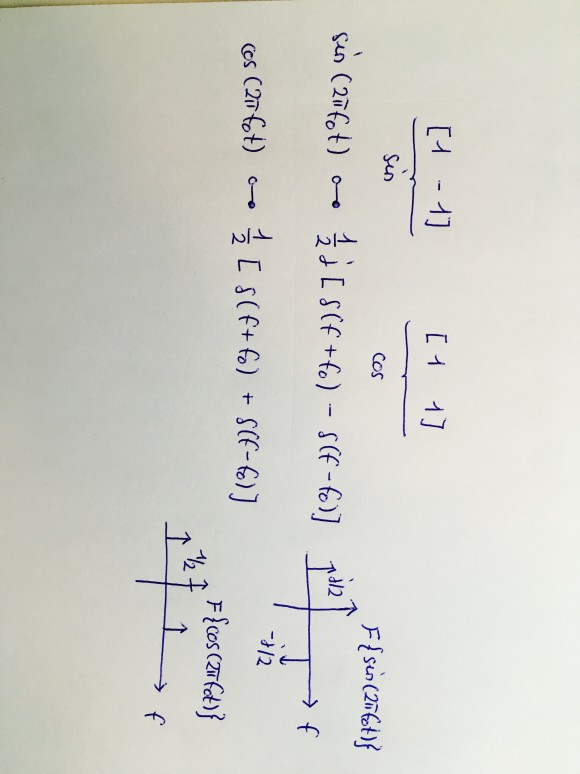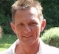## Digital Image ProcessingStarted by 5 years ago3 replieslatest reply 5 years ago131 views

Hey guys,

I am currently trying to solve the following two questions in preparation of my digital image processing exam. I thought, maybe you can try and guide me to the correct solution, since I am stuck.So, the first question: I know the answer must be related to "Aliasing" or "Leakage" or maybe "Moirè". Leakage results in frequency domain if the spatial domain is multiplied by a rectangle function. This is virtually always the case, since the spatial domain cannot be infinite. Multiplying by a rectangle function in spatial domain then results in a convolution with a sinc function in frequency domain and this will lead to a smudge effect. But I do not see how this is inherent to the given input signal. Aliasing results from overlapping of the periodic repetitions in spectral domain. I am really not sure about the DFT of a sine function. I mean, is this input signal even discrete? I mean, in a DFT don't we usually consider samples of a DTFT, meaning we have .. I don't know, I am lost, I am confused by the sample frequency appearing in my signal. But usually most transforms of a sine are related to two shifted Diracs which do not have any bandwidth and, therefore, cannot overlap. I think this input signal is related to the Moirè effect. I don't understand this effect very well, but it is some kind of optical illusion that occurs when two sine waves of similar frequencies are added. Is this happenning here?

Now, coming to the second question: [1 -1] is a vector corresponding to a sine and [1 1] is a vector corresponding to a cosine. What is meant by plotting the magnitudes of these Fourier transforms ?

I mean, literally, all I can think of is this simple relationship:[ - ]A quick look to the s(nx) definition makes me assume that in the spectral domain you see not a single line as would be ideal for a sine response in the cont.math world.
Instead because of the fractional value of 3.5 you'd see the line dispersed to the two neighbor bins, each with half the amplitude as you would expect in cont.FFT.

For the second question: I would understand a pulse of (1,-1) as being value -1 as the sample with number one, all other samples having value 0. (1,1) then means a positive pulse with value 1, again only at point of first sample.

Hope this is a correct interpretation, and that it helps you ...
Bernhard

[ - ]Well let us try:

Fs = 1;

N = 1024;

x = sin(2*pi*3.5*Fs*(0:N-1)/N);

y = fft(x,N);

plot(20*log10(abs(y)));

[ - ]